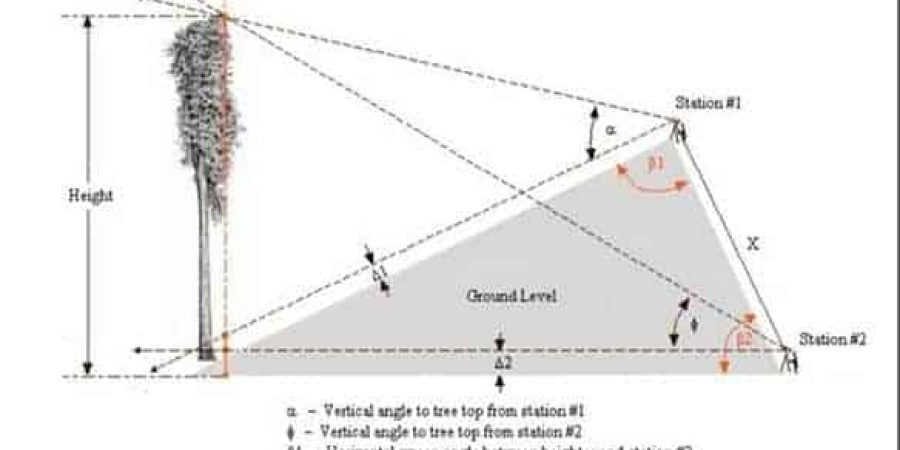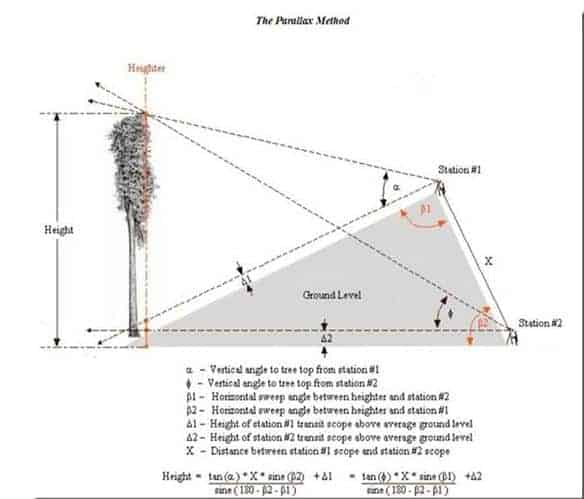316-722-2348

M-F: 8am to 5pm

### Emergency Service## Using the Parallax Method for Measuring Tree HeightThe Parallax method has been used for decades to measure the height of a tree. The image below shows the Parallax method for measuring tree height as developed by Michael Taylor. Michael Taylor has presented versions of this method in the past. Michael’s diagram is the clearest.

This method has received little attention from NTS members, because is suspect that it is not intuitive. Also, angle and distance errors have a magnified effect. Below I have created steps for measuring a tree height using the Parallax Method.

## How to Measure Tree Height

1. Establish a baseline, from the ends of which, you can see the target

2. Mark the ends of the baseline as stations S1 and S2
3. Measure the baseline as X1 and its slope angle as Z from S1 to S2
4. From S1 sight S2 and take its azimuth AZ1,1
5. Then sight taregt T from S1 and take T’s azimuth AZ1,2
6. Compute angle a1 from the two azimuths
7. From S2 sight S1 and take its azimuth AZ2,18. Sight taregt T from S2 and take T’s azimuth AZ2,2
9. Compute angle a2 from the two azimuths
10. Compute angle c
11. Compute D1 and D2
12. From S1, take angle A1 to T in vertical plane
13. From S2, take angle A2 to T in vertical plane
using formulas below
15. H1 and H2 are determinations of height
of T above S1 and S2 resepctively
16. Compute H3, the elevation of S1
above/below S2 so that H1 and H2 can be compared

17. If H1 differs from H2 +/- H3 by more than a specified amount,
e.g. +/- 1.5 feet, the measurements should be retaken.
18. Note that the above process is implemented in the table below.

### Using the Parallax Method for Measuring Tree Height

The Parallax method has been used for decades to measure the height of a tree. The image below shows the Parallax method for measuring tree

### Tree Safety

Tree Safety – Always In Your Best Interest Tree workers suffer from injuries at a rate three times the national average for the blue-collar workforce.